What does it do?

It returns a cell´s value from within a table/range

Formula breakdown:

=INDEX(array, row_num, [column_num])

What it means:

=INDEX(from this table/range, return me this row number, [and return me this column number])

The INDEX function in Excel returns a cell´s values from within a table/array.

It works like a map, so you have to select a range (table/array) and tell it to return you the coordinates (Row & Column numbers).

So if you want to return values from a Price List or large data set, then your INDEX function is your savior.

We want to get the price of a laptop in 2014 and 2015 based on price table.

STEP 1: We need to enter the INDEX function in a blank cell:

## =INDEX(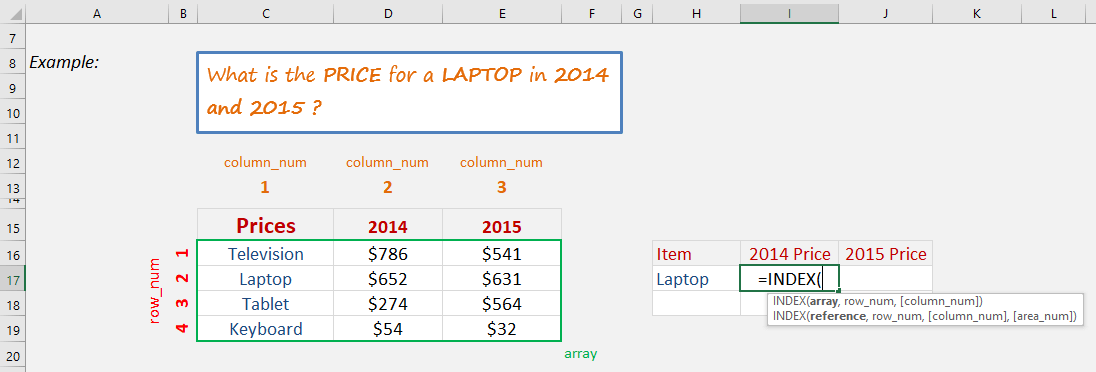STEP 2: The INDEX arguments for the 2014 laptop price:

## Array

What is the table of source data?

We need to select the pricing table here.

## =INDEX(C16:E19,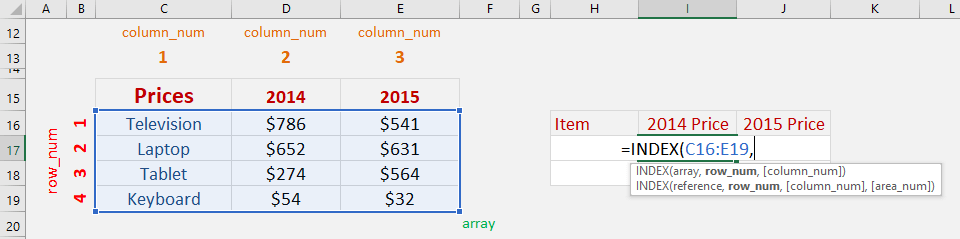## Row_num

What row number contains the data?

Since we want the laptop, it’s on row #2

## =INDEX(C16:E19, 2,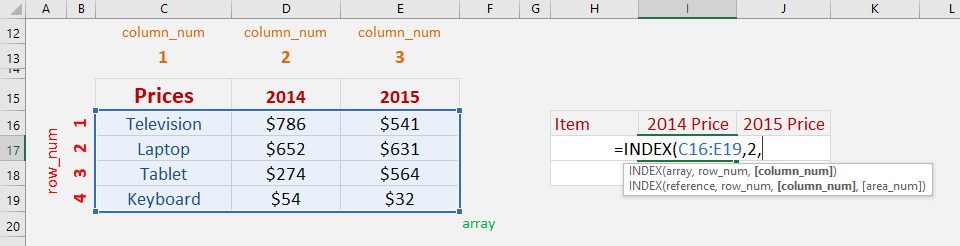## Column_num

What column number contains the data?

Since we want the price for the year 2014, it’s on column #2

## =INDEX(C16:E19, 2, 2)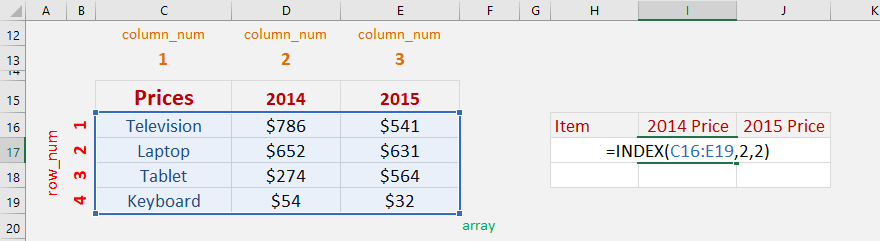STEP 3: The INDEX arguments for the 2015 laptop price:

## Array

What is the table of source data?

We need to select the pricing table here.

## =INDEX(C16:E19,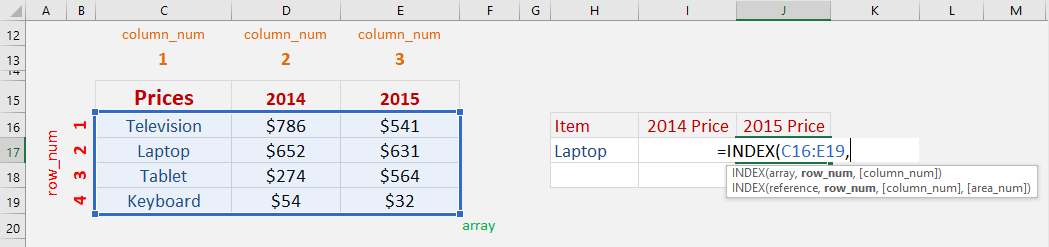## Row_num

What row number contains the data?

Since we want the laptop, it’s on row #2

## =INDEX(C16:E19, 2,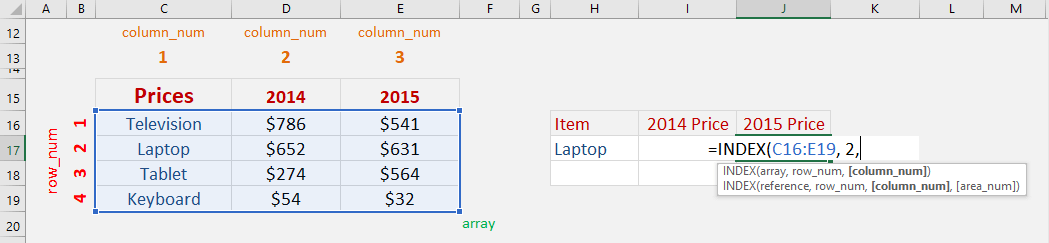## Column_num

What column number contains the data?

Since we want the price for the year 2015, it’s on column #3

## =INDEX(C16:E19, 2, 3)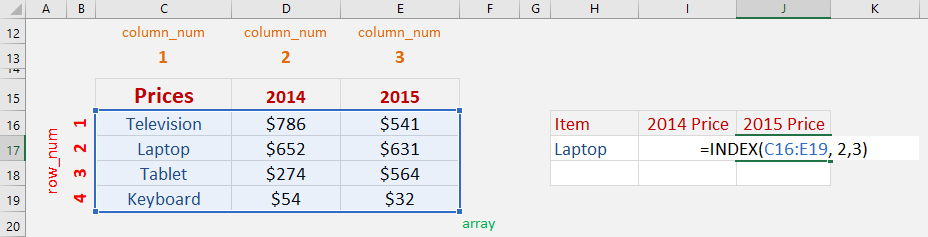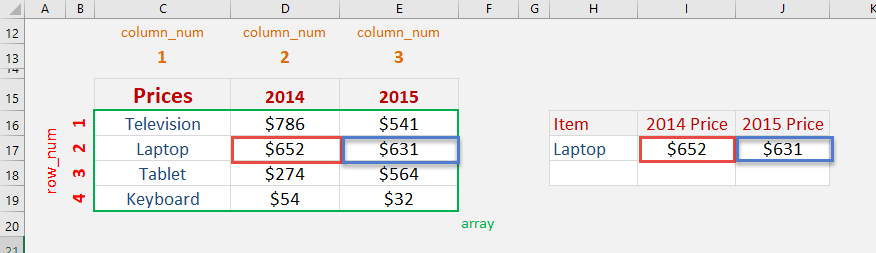How to Use the Index Formula in Excel